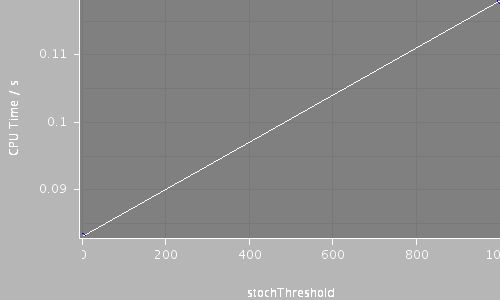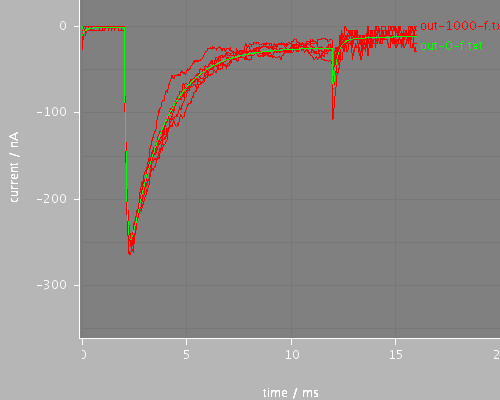## step-onset-avg (run-onset-avg.xml)

Voltage clamp step onset behavior for continuous and stochastic channels. The step begins at 1.985ms and the timestep is 0.02ms. This uses the average evaluation method for the step, (stepStyle = average in the voltage clamp) so the effect is as if the onset occurred in two steps: one step beginning at 1.98 to the average of the hold and step values over the step (about 0mv in this case - three quarters of the way from -80mV to 30mV) and the next step beginning at 2.0ms with potential fixed at the step value (30mV). In this case, the use of the average produces an artifact in the results: the step to 0mV opens fewer channels but gives a larger current (larger driving force at 0mV than at 30mV) than a step to 30mV would. The following step takes it to the normal current at that stage of the step, but because the previous current was so big, the transition between steps appears flatter, giving the appearance of a kink in the current. This fugure should be compared with the file run-onset, which does the same calculation but with stepStyle = midpoint for the clamp.

Total CPU time 0.2010 seconds; at 16:38:55 Fri 28 Sep 2007

 Compartments Stochasticchannels / cpmts Continuouschannels / cpmts time/ms timestep/ms stochThreshold CPU Time / s 1 0 / 0 201 / 1 16.0 0.02 0 0.083 1 201 / 1 0 / 0 16.0 0.02 1000 0.118### Predefined views

#### whole#### start## All files

 Model Preprocessed Outupt data Reference data etc run-onset-avg.xml cell.xml membrane.xml environment.xml recording-short-avg.xml na1.xml summary.xml view.xml out-0-fdata.txt out-1000-fdata.txt out-0-f.dat out-0-f.txt out-1000-f.dat out-1000-f.txt step-onset-avg.jar

## Model

Archive file of the complete model: step-onset-avg.jar

### run-onset-avg.xml

```<PSICSRun timeStep="0.02ms" runTime="16ms" startPotential="-65mV"
morphology="cell"
environment="environment"
properties="membrane"
access="recording-short-avg"
stochThreshold="0"
repeats="20">
<StructureDiscretization baseElementSize="3um"/>

<RunSet vary="stochThreshold" values="[0, 1000]"
filepattern="out-\$.txt">
</RunSet>

Voltage clamp step onset behavior for continuous and stochastic channels. The step begins
at 1.985ms and the timestep is 0.02ms. This uses the average evaluation method for the step,
(stepStyle="average" in the voltage clamp)
so the effect is as if the onset occurred in two steps: one step beginning at 1.98 to the
average of the hold and step values over the step (about 0mv in this case - three quarters of the way from -80mV to 30mV)
and the next step beginning at 2.0ms with potential fixed at the step value (30mV).
In this case, the use of the average produces an artifact in the results: the step to 0mV
opens fewer channels but gives a larger current (larger driving force at 0mV than at 30mV) than
a step to 30mV would. The following step takes it to the normal current at that stage of the step, but
because the previous current was so big, the transition between steps appears flatter, giving the
appearance of a kink in the current.
This fugure should be compared with
the file run-onset, which does the same calculation but with stepStyle="midpoint" for the clamp.

<ViewConfig>
<LineGraph width="500" height="400">
<XAxis min="0" max="250" label="time / ms"/>
<YAxis min="-80" max="60" label="current / nA"/>

<LineSet file="out-1000-f.txt" color="red" maxshow="6"/>
<LineSet file="out-0-f.txt" color="green" maxshow="2"/>

<View id="whole" xmin="0." xmax="20." ymin="-360." ymax="30."/>
<View id="start" xmin="1.93" xmax="2.15" ymin="-260." ymax="30."/>
</LineGraph>

</ViewConfig>

</PSICSRun>
```

### cell.xml

```<CellMorphology id="cell">

<Point id="p0" x="0" y="0" z="0" r="1."/>

</CellMorphology>
```

### membrane.xml

```<CellProperties id="membrane"
cytoplasmResistivity="100ohm_cm"
membraneCapacitance="1uF_per_cm2">

<ChannelPopulation id="napop" channel="na1" density="16per_um2"/>

</CellProperties>
```

### environment.xml

```<CellEnvironment id="environment">
<Ion id="Na" name="Sodium" reversalPotential="80mV"/>
<Ion id="K" name="Potassium" reversalPotential="-80mV"/>
</CellEnvironment>
```

### recording-short-avg.xml

```<Access id="recording-short-avg" saveInterval="0.05ms">

<VoltageClamp at="p0" lineColor="red" hold="-80.0mV" stepValue="average">
<VoltagePulse id="vstep" start="1.985ms" to="30mV"
duration="10ms"/>
</VoltageClamp>

</Access>
```

### na1.xml

```<KSChannel id="na1" gSingle="30pS" permeantIon="Na">
<ClosedState id="c1"/>
<OpenState id="o1"/>
<ClosedState id="c2"/>

<VHalfTransition from="c1" to="o1" vHalf = "-35mV" z="4.5e" gamma="0.8" tau="0.55ms" tauMin="0.1ms"/>

<VHalfTransition from="o1" to="c2" vHalf = "-70mV" z="1.1e" gamma="0.90" tau="12.0ms" tauMin="1.5ms"/>

</KSChannel>
```